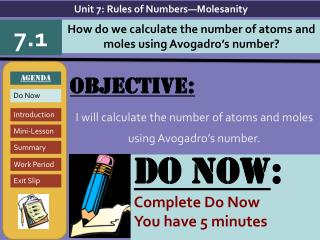Download PresentationObjective: I will calculate the number of atoms and moles using Avogadro’s number.

# Objective: I will calculate the number of atoms and moles using Avogadro’s number. - PowerPoint PPT PresentationDownload Presentation## Objective: I will calculate the number of atoms and moles using Avogadro’s number.

- - - - - - - - - - - - - - - - - - - - - - - - - - - E N D - - - - - - - - - - - - - - - - - - - - - - - - - - -
##### Presentation Transcript

1. Unit 7: Rules of Numbers—Molesanity 7.1 How do we calculate the number of atoms and moles using Avogadro’s number? AGENDA Do Now Introduction Mini-Lesson Summary Work Period Exit Slip Objective: I will calculate the number of atoms and moles using Avogadro’s number. DO NOW: Complete Do Now You have 5 minutes

2. Unit 7: Rules of Numbers—Molesanity How do we calculate the number of atoms and moles using Avogadro’s number? 7.1 AGENDA Introduction Mini-Lesson Summary Do Now Work Period Exit Slip Real-world connection Dozen eggs Vocabulary Mole, Avogadro’s number

3. Unit 7: Rules of Numbers—Molesanity How do we calculate the number of atoms and moles using Avogadro’s number? 7.1 AGENDA Introduction Mini-Lesson Summary Do Now Work Period Exit Slip Announcements Review sheets must be turned in today or past due Packet #15 due today Regents prep during 5th period today Peer Leader applications due tomorrow

4. Unit 7: Rules of Numbers—Molesanity How do we calculate the number of atoms and moles using Avogadro’s number? 7.1 AGENDA Introduction Mini-Lesson Summary Do Now Work Period Exit Slip Do now

5. Unit 7: Rules of Numbers—Molesanity How do we calculate the number of atoms and moles using Avogadro’s number? 7.1 • Catalyzing thoughts • Mr. Walters wants to organize a pizza party for those students show a 10% improvement in grades for the next quarter. Two pizzas will need to be ordered for each group of 10 students. • How many pizzas would have to be ordered for 20 students? • How many pizzas would have to be ordered for 30 students? Work Period Summary Mini-Lesson Exit Slip Do Now Introduction

6. Unit 7: Rules of Numbers—Molesanity How do we calculate the number of atoms and moles using Avogadro’s number? 7.1 • Catalyzing thoughts • How about making that pizza: • To make 1 pizza, it requires 50 pepperonis, 2 pounds of cheese and 1 pound of flour. • 50 pepperonis + 2 pounds of cheese + 1 pound of flour = 1 pizza • If you had 150 pepperonis, how many pizzas could you make? Assuming you had an unlimited supply of the other ingredients. • If you had 4 pounds of cheese, how many pizzas could you make Work Period Summary Mini-Lesson Exit Slip Do Now Introduction

7. Unit 7: Rules of Numbers—Molesanity How do we calculate the number of atoms and moles using Avogadro’s number? 7.1 • The mole Work Period Summary Mini-Lesson Exit Slip Do Now Introduction

8. Unit 7: Rules of Numbers—Molesanity How do we calculate the number of atoms and moles using Avogadro’s number? 7.1 • A chuckle or two… Work Period Summary Mini-Lesson Exit Slip Do Now Introduction

9. Unit 7: Rules of Numbers—Molesanity How do we calculate the number of atoms and moles using Avogadro’s number? 7.1 • Moles  particles conversions Work Period Summary Mini-Lesson Exit Slip Do Now Introduction

10. Unit 7: Rules of Numbers—Molesanity How do we calculate the number of atoms and moles using Avogadro’s number? 7.1 • You try! • Cross out page 5 • Go to page 7 • Then go back to page 6 Work Period Summary Mini-Lesson Exit Slip Do Now Introduction

11. Unit 7: Rules of Numbers—Molesanity How do we calculate the number of atoms and moles using Avogadro’s number? 7.1 • You try! • Calculate the number of moles in each of the following: • How many moles of radon do 9.77 x 1022 atoms equal? • Find the moles in 2.44 x 1025 molecules of CaS. • Find the number of moles present in 6.57 x 1029 of LiF Work Period Summary Mini-Lesson Exit Slip Do Now Introduction

12. Unit 7: Rules of Numbers—Molesanity How do we calculate the number of atoms and moles using Avogadro’s number? 7.1 • Questions to try! • Calculate the number of atoms in each of the following: • a. 1 mole KI d. 1.3 moles Fe2O3 • b. 3.2 moles He e. 0.75 moles NaNO3 • c. 0.023 moles KOH f. 0.23 moles H2O Work Period Summary Mini-Lesson Exit Slip Do Now Introduction

13. Unit 7: Rules of Numbers—Molesanity How do we calculate the number of atoms and moles using Avogadro’s number? 7.1 Work period Complete pages 6-7 Work Period Exit Slip Introduction Do Now Mini-Lesson Summary

14. Unit 7: Rules of Numbers—Molesanity How do we calculate the number of atoms and moles using Avogadro’s number? 7.1 HOMEWORK: Complete rest of 7.1 in the packet for homework Work Period Exit Slip Introduction Do Now Mini-Lesson Summary

15. Unit 7: Rules of Numbers—Molesanity How do we calculate the number of atoms and moles using Avogadro’s number? 7.1 Exit Slip Make sure you get your trackers checked! Exit Slip Introduction Do Now Mini-Lesson Summary Work Period